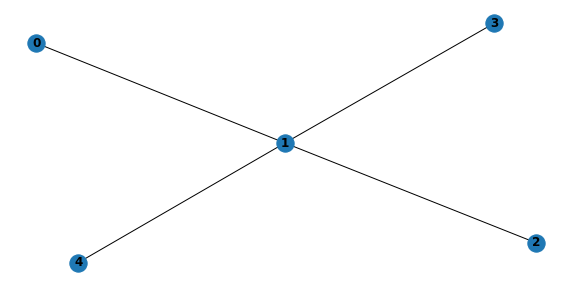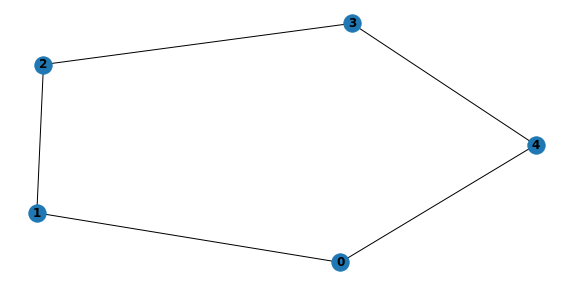# 1. Generating Random Bayesian Network

This notebook shows how to generate singly- and multi-connected Bayesian Belief Networks (BBNs). The algorithms are taken directly from here. When generating a BBN, you have to generate

• the structure, which is a directed acyclic graph (DAG), and

• the parameters, which are local probability models.

In this notebook, the parameters are assumed to take on the Dirichlet-Multinomial distribution. If you are wondering, a singly-connected BBN is one when, ignoring the directions of the edges in the DAG, there is at most one path between any two nodes. A multi-connected BBN is one that is not singly-connected (it is defined by the negation of a singly-connected BBN). The BBNs generated using this approach gaurantees the distribution of the BBNs are uniformly distributed (look at the paper for proofs, details, and benefits of having this property).

## 1.1. Generate the structure

Here, we generate the DAGs of a singly- and multi-connected BBNs. Note that when we visualize the DAGs, we do so by converting it to an undirected graph only because the layout algorithm is more aesthetically pleasing.

:

import warnings
import networkx as nx
import numpy as np
import json
import matplotlib.pyplot as plt
from networkx.algorithms.dag import is_directed_acyclic_graph
from networkx.algorithms.shortest_paths.generic import shortest_path

np.random.seed(37)

def get_simple_ordered_tree(n):
"""
Generates a simple-ordered tree. The tree is just a
directed acyclic graph of n nodes with the structure
0 --> 1 --> .... --> n.
"""
g = nx.DiGraph()

for i in range(n):

for i in range(n - 1):
return g

def convert_to_undirected_graph(g):
"""
Converts a directed acyclic graph (DAG) to an undirected graph.
We need to convert a DAG to an undirected one to use
some API calls to operate over the undirected graph. For example,
in checking for connectedness of a graph, the API has a method
to check for connectedness of an undirected graph, but not a
DAG.
"""
u = nx.Graph()
for n in g.nodes:
for e in g.edges:
return u

def is_connected(g):
"""
Checks if a the directed acyclic graph is connected.
"""
u = convert_to_undirected_graph(g)
return nx.is_connected(u)

def get_random_node_pair(n):
"""
Randomly generates a pair of nodes.
"""
i = np.random.randint(0, n)
j = i
while j == i:
j = np.random.randint(0, n)
return i, j

def edge_exists(i, j, g):
"""
Checks if the edge i --> j exists in the graph, g.
"""
return j in list(g.successors(i))

def del_edge(i, j, g):
"""
Deletes the edge i --> j in the graph, g. The edge is only
deleted if this removal does NOT cause the graph to be
disconnected.
"""
if g.has_edge(i, j) is True:
g.remove_edge(i, j)

if is_connected(g) is False:

"""
Adds an edge i --> j to the graph, g. The edge is only
cycles.
"""
if is_directed_acyclic_graph(g) is False:
g.remove_edge(i, j)

def find_predecessor(i, j, g):
"""
Finds a predecessor, k, in the path between two nodes, i and j,
in the graph, g. We assume g is connected, and there is a
path between i and j (ignoring the direction of the edges).
We want to find a k, that is a parent of j, that is in
the path between i and j. In some cases, we may not find
such a k.
"""
parents = list(g.predecessors(j))
u = convert_to_undirected_graph(g)
for pa in parents:
try:
path = shortest_path(u, pa, i)
return pa
except:
pass
return None

def generate_multi_connected_structure(n, max_iter=10):
"""
Generates a multi-connected directed acyclic graph.
"""
g = get_simple_ordered_tree(n)
for it in range(max_iter):
i, j = get_random_node_pair(n)
if g.has_edge(i, j) is True:
del_edge(i, j, g)
else:
return g

def generate_singly_structure(n, max_iter=10):
"""
Generates a singly-connected directed acyclic graph.
"""
g = get_simple_ordered_tree(n)

counter = 0
for it in range(max_iter):
i, j = get_random_node_pair(n)
if g.has_edge(i, j) is True or g.has_edge(j, i) is True:
pass
else:
p = np.random.random()
k = find_predecessor(i, j, g)
if k is not None:
g.remove_edge(k, j)
if p < 0.5:
else:

if is_connected(g) is False:

if p < 0.5:
g.remove_edge(j, i)
else:
g.remove_edge(i, j)
return g


### 1.1.1. Generate DAG for singly-connected BBN

:

with warnings.catch_warnings(record=True):
s = generate_singly_structure(5, 1000)
plt.figure(figsize=(10, 5))
plt.subplot(111)
nx.draw(convert_to_undirected_graph(s), with_labels=True, font_weight='bold')### 1.1.2. Generate DAG for multi-connected BBN

:

with warnings.catch_warnings(record=True):
m = generate_multi_connected_structure(5, 10)
plt.figure(figsize=(10, 5))
plt.subplot(111)
nx.draw(convert_to_undirected_graph(m), with_labels=True, font_weight='bold')## 1.2. Generate the parameters

Here, we generate parameters for the BBNs.

:

from scipy.stats import dirichlet, multinomial

def generate_num_values(n, max_values=2):
"""
For each node, i, in the nodes, n, determine the number of values
the node (or equivalently, variable) has. Every node/variable in a
Bayesian Network should have 2 or more values. This generates
the number of values each variable will have. Each number will be
sampled uniformly.
"""
return np.array([max(np.random.randint(0, max_values) + 1, 2) for _ in range(n)])

def generate_alphas(n, max_alpha=10):
"""
Generate random number for the alpha's (the hyperparameters).
Each number will be in the range [1, max_alpha]. Each number will
be sampled uniformly.
"""
return [np.random.randint(1, max_alpha + 1) for i in range(n)]

def sample_dirichlet(n, max_alpha=10):
"""
Samples from the Dirichlet distribution to a produce
a probability vector of length n. The sum of each probability
in the probability vector should sum to 1.
"""
return np.array(dirichlet.rvs(generate_alphas(n, max_alpha)))

def get_num_parent_instantiations(parents, num_values):
num_pa_instantiations = 1
for pa in parents:
num_pa_values = num_values[pa]
num_pa_instantiations *= num_pa_values
return num_pa_instantiations

def generate_dirichlet_parameters(i, parents, num_values, max_alpha=10):
"""
Randomly and uniformly generate parameters for a node i. A matrix
of parameters will be returned. The matrix will represent the
condtional probability table of the node i. The matrix will have
the dimensions m (rows) by n (columns), m x n, where m is the
product of the domain sizes of the parents, and n is the domain
size of the node. The domain size is just the number of values
that a node (variable) has, which should always be greater than
or equal to 2.
"""
num_pa_instantiations = get_num_parent_instantiations(parents, num_values)

n = num_values[i]
cpt = []
for pa_instantiation in range(num_pa_instantiations):
probs = sample_dirichlet(n, max_alpha)
cpt.append(probs)
return np.array(cpt)

def generate_parameters(g, max_values=2, max_alpha=10):
"""
Generates parameters for each node in the graph, g.
A dictionary indexed by the node's id will give its
(sampled) parameters and its parents.
"""
num_nodes = len(list(g.nodes))
num_values = generate_num_values(num_nodes, max_values)
g_params = {}
for i in g.nodes:
parents = list(g.predecessors(i))
params = generate_dirichlet_parameters(i, parents, num_values, max_alpha)
g_params[i] = {
'parents': parents,
'params': params,
'shape': [get_num_parent_instantiations(parents, num_values), num_values[i]]
}
return g_params


### 1.2.1. Generate parameters for singly-connected BBN

:

s_params = generate_parameters(s)
print(s_params)

{0: {'parents': [], 'params': array([[0.74174228, 0.25825772]]), 'shape': [1, 2]}, 1: {'parents': [2, 3, 0, 4], 'params': array([[0.50086958, 0.49913042],
[0.02647713, 0.97352287],
[0.42054028, 0.57945972],
[0.85369846, 0.14630154],
[0.22294388, 0.77705612],
[0.36867171, 0.63132829],
[0.86317661, 0.13682339],
[0.12457256, 0.87542744],
[0.50873009, 0.49126991],
[0.82929381, 0.17070619],
[0.67980646, 0.32019354],
[0.86189091, 0.13810909],
[0.8995967 , 0.1004033 ],
[0.53483643, 0.46516357],
[0.52939964, 0.47060036],
[0.83144941, 0.16855059]]), 'shape': [16, 2]}, 2: {'parents': [], 'params': array([[0.42051707, 0.57948293]]), 'shape': [1, 2]}, 3: {'parents': [], 'params': array([[0.80468897, 0.19531103]]), 'shape': [1, 2]}, 4: {'parents': [], 'params': array([[0.67431296, 0.32568704]]), 'shape': [1, 2]}}


### 1.2.2. Generate parameters for muti-connected BBN

:

m_params = generate_parameters(m)
print(m_params)

{0: {'parents': [], 'params': array([[0.27733808, 0.72266192]]), 'shape': [1, 2]}, 1: {'parents': , 'params': array([[0.50940174, 0.49059826],
[0.99785858, 0.00214142]]), 'shape': [2, 2]}, 2: {'parents': [1, 3], 'params': array([[0.33727417, 0.66272583],
[0.79651078, 0.20348922],
[0.40652547, 0.59347453],
[0.88763038, 0.11236962]]), 'shape': [4, 2]}, 3: {'parents': [], 'params': array([[0.85069727, 0.14930273]]), 'shape': [1, 2]}, 4: {'parents': [3, 0], 'params': array([[0.68777784, 0.31222216],
[0.4609832 , 0.5390168 ],
[0.46319254, 0.53680746],
[0.10677866, 0.89322134]]), 'shape': [4, 2]}}


## 1.3. Persist (save) the Bayesian Belief Network

Here, we show how to save the BBN (the DAG and parameters). Note that we save it to a JSON file format. There are simply too many formats for BBNs, but the JSON format here has all the information you need to convert it to any other format.

:

def to_json(g, params, pretty=True):
to_int_arr = lambda arr: [int(item) for item in arr]
j = {}

j['nodes'] = list(g.nodes)
j['edges'] = [{'pa': e, 'ch': e} for e in g.edges]
j['parameters'] = [{'node': k,
'params': list(v['params'].flatten()),
'shape': to_int_arr(v['shape'])}
for k, v in params.items()]
if pretty:
return json.dumps(j, indent=2, sort_keys=False)
return json.dumps(j)


### 1.3.1. Persist singly-connected BBN

:

s_json = to_json(s, s_params)
print(s_json)
with open('./output/singly-connected.json', 'w') as fhandle:
fhandle.write(to_json(s, s_params, pretty=True))

{
"nodes": [
0,
1,
2,
3,
4
],
"edges": [
{
"pa": 0,
"ch": 1
},
{
"pa": 2,
"ch": 1
},
{
"pa": 3,
"ch": 1
},
{
"pa": 4,
"ch": 1
}
],
"parameters": [
{
"node": 0,
"params": [
0.7417422803682197,
0.25825771963178046
],
"shape": [
1,
2
]
},
{
"node": 1,
"params": [
0.5008695756264835,
0.4991304243735164,
0.026477131905188734,
0.9735228680948113,
0.42054028327937387,
0.579459716720626,
0.8536984622587769,
0.14630153774122318,
0.22294387680535926,
0.7770561231946408,
0.3686717093409431,
0.6313282906590567,
0.8631766076552874,
0.13682339234471266,
0.1245725606108167,
0.8754274393891833,
0.5087300903407761,
0.49126990965922374,
0.8292938143560679,
0.1707061856439322,
0.6798064596245497,
0.32019354037545045,
0.8618909056718044,
0.13810909432819546,
0.8995966961793681,
0.10040330382063184,
0.5348364275608116,
0.4651635724391883,
0.5293996424146508,
0.47060035758534935,
0.8314494130139252,
0.16855058698607464
],
"shape": [
16,
2
]
},
{
"node": 2,
"params": [
0.42051706936960864,
0.5794829306303914
],
"shape": [
1,
2
]
},
{
"node": 3,
"params": [
0.8046889692800407,
0.19531103071995928
],
"shape": [
1,
2
]
},
{
"node": 4,
"params": [
0.6743129580736985,
0.3256870419263015
],
"shape": [
1,
2
]
}
]
}


### 1.3.2. Persist multi-connected BBN

:

m_json = to_json(m, m_params)
print(m_json)
with open('./output/multi-connected.json', 'w') as fhandle:
fhandle.write(to_json(m, m_params, pretty=True))

{
"nodes": [
0,
1,
2,
3,
4
],
"edges": [
{
"pa": 0,
"ch": 1
},
{
"pa": 0,
"ch": 4
},
{
"pa": 1,
"ch": 2
},
{
"pa": 3,
"ch": 4
},
{
"pa": 3,
"ch": 2
}
],
"parameters": [
{
"node": 0,
"params": [
0.2773380778096063,
0.7226619221903937
],
"shape": [
1,
2
]
},
{
"node": 1,
"params": [
0.5094017382812316,
0.4905982617187683,
0.9978585783502765,
0.002141421649723624
],
"shape": [
2,
2
]
},
{
"node": 2,
"params": [
0.3372741656699353,
0.6627258343300647,
0.7965107759562755,
0.20348922404372444,
0.40652547264228284,
0.5934745273577172,
0.8876303836604758,
0.11236961633952422
],
"shape": [
4,
2
]
},
{
"node": 3,
"params": [
0.8506972724905534,
0.14930272750944656
],
"shape": [
1,
2
]
},
{
"node": 4,
"params": [
0.6877778356657721,
0.31222216433422784,
0.4609831964438165,
0.5390168035561835,
0.4631925375186775,
0.5368074624813227,
0.10677865814938657,
0.8932213418506135
],
"shape": [
4,
2
]
}
]
}


## 1.4. All-in-one (AIO) example

Here’s a simple AIO example of generating a singly-connected BBN and its corresponding JSON.

:

g = generate_singly_structure(5, 1000)
p = generate_parameters(g)
j = to_json(g, p)